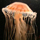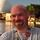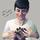## General Question# Could someone explain this trigonometry question for me?

Asked by dotlin (419) July 27th, 2010

http://yfrog.com/5ctrigj
h=d tanθ1 tanθ2 / tan θ2 -tanθ1

I show can’t see how this works out h.

Observing members: 0Composing members: 0## 8 AnswersSo I’ll explain what I get.

lets replace h:o and d:a tan= o/a but of course we don’t have the full length of a so by rearranging it to o=tanθ a isn’t the right answer so far.

I don’t get why tanθ2 / tan θ2 -tanθ1 is there.

dotlin (419)“Great Answer” (0) Flag as…Writing out equations is tricky on a keyboard. Can you clarify it a little bit, using explicit parentheses and multiplication signs, so that we can be sure we’re seeing the same equations you are?

MrItty (17376)“Great Answer” (0) Flag as…I got it in a really roundabout way. I’m going to label “a” as the section of the bottom line that’s to the left of D.

tanθ1 = h/(a+D) and tanθ2 = h/a
I then solved for a in each equation
a = (h – D*tanθ1)/tanθ1 and a = h/tanθ2
Then I set the two a’s equal to each other
h/tanθ2 = (h – D*tanθ1)/tanθ1
Cross-multiply…
h*tanθ1 = h*tanθ2 – D*tanθ1*tanθ2
Put the h’s on the same side of the equation
h*tanθ1 – h*tanθ2 = D*tanθ1*tanθ2
Take out the common factor
h*(tanθ1 – tanθ2) = D*tanθ1*tanθ2
Then solve for h
h = (D*tanθ1*tanθ2)/(tanθ1 – tanθ2)
Voila!

Mariah (25831)“Great Answer” (1) Flag as…Oh, shoot. This isn’t homework, is it? I probably shouldn’t have helped you quite that much if it was…

Mariah (25831)“Great Answer” (0) Flag as…No school is out just wanted to know.

dotlin (419)“Great Answer” (0) Flag as…@Mariah
I’m just having struggle imagining a = (h – D*tanθ1). a = h/tanθ2 is basic trig but a = (h – D*tanθ1) doesn’t feel instinctive at all.

Try and explain that formula in words so it may help me then.

dotlin (419)“Great Answer” (0) Flag as…Yes everything else seems fine apart from not instinctively getting that, thanks so much so far

dotlin (419)“Great Answer” (0) Flag as…Start with tanθ1 = h/(a+D)
Multiply both sides by a+D: (a+D)*tanθ1 = h
Distribute: a*tanθ1 + D*tanθ1 = h
Subtract: a*tanθ1 = h – D*tanθ1
Divide: a = (h – D*tanθ1)/tanθ1

Mariah (25831)“Great Answer” (1) Flag as…## Answer this questionor

#### Join

to answer.

This question is in the General Section. Responses must be helpful and on-topic.

Your answer will be saved while you login or join.
Have a question? Ask Fluther!
What do you know more about?
or
Knowledge Networking @ Fluther